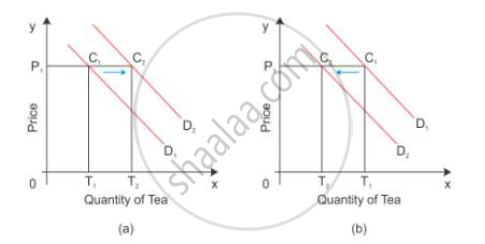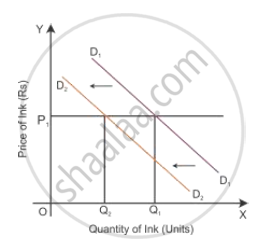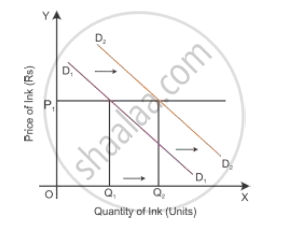# Prices of Other Goods and Demand for the Given Good. - Economics

Prices of other goods and demand for the given good.

#### Solution

Price of Other Goods and Demand for the given Good

Demand for a commodity in relation to price of the substitute good

When the price of one good falls, it becomes cheaper in relation to another good. As a result, one good is substituted for the other good such as coffee and tea. Assume tea and coffee are two substitute goods. D1 is the demand curve for the demand of tea in diagram (a).

Increase in price of substitute good:-

When the price of tea is OP1, the quantity demanded is OT1 as shown in diagram (a). If there is an increase in the price of the substitute good coffee, then the demand curve for tea shifts to the right. Now, the consumer is willing to buy P1C2 quantity of tea which is equal to OT2. Greater the purchase of a commodity at its constant price points to a situation of increase or forward shift in the demand curve. The consumer demand curve shifts from D1 to D2, consuming more of tea even when its price is constant.Decrease in price of substitute good:-

When there is a decrease in the price of the substitute good coffee, the demand curve for tea shifts to the left even when its price is constant. When the price of tea is OP1, the quantity demanded is OT1 as shown in the diagram (b). Now, the consumer is willing to buy P1C2 quantity of tea which is equal to OT2. Thus, the consumer shifts from D1 to D2, consuming less of tea even when the price of tea is constant. This is a situation of backward
shift in the demand curve.

Demand for a commodity in relation to price of the complementary good

Complementary goods are purchased jointly such as ink and ink pens.

Increase in price of complementary good:-

If there is an increase in the price of a good, then the demand for another good will decline. So the demand curve shifts parallel to the left, i.e. from D1D1 to D2D2.Decrease in price of complementary good:-

If there is a decrease in the price of a good, then the demand for another good will increase. So the demand curve shifts parallel to the right, i.e. from D1D1 to D2D2.Concept: Demand Curve and Its Slope
Is there an error in this question or solution?